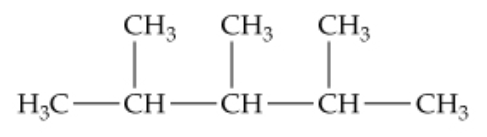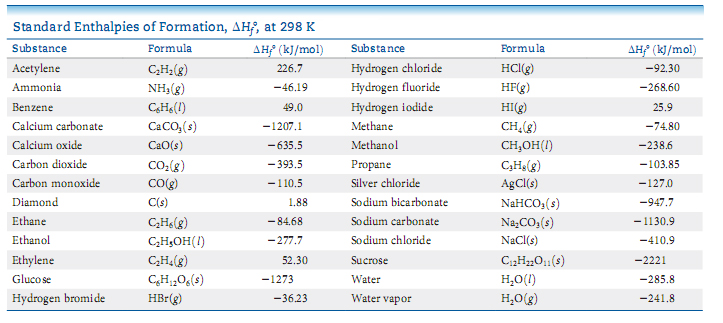# Problem: Gasoline is composed primarily of hydrocarbons, including many with eight carbon atoms, called octanes. One of the cleanest-burning octanes is a compound called 2,3,4-trimethylpentane, which has the structural formula shown.The complete combustion of one mole of this compound to CO2(g) and H2O(g) leads to ΔH˚ = –5064.9 kJ/mol{ m kJ/mol}. By using the information in this problem and data given below, calculate ΔH˚f for 2,3,4-trimethylpentane.

###### FREE Expert Solution

C8H18(l) + 25/2 O2(g) → 8 CO2(g) and 9 H2O(g)

Note that we need to multiply each ΔH˚f by the stoichiometric coefficient since ΔH˚f is in kJ/mol.

Also, note that ΔH˚f for elements in their standard state is 0.

84% (262 ratings)###### Problem Details

Gasoline is composed primarily of hydrocarbons, including many with eight carbon atoms, called octanes. One of the cleanest-burning octanes is a compound called 2,3,4-trimethylpentane, which has the structural formula shown.The complete combustion of one mole of this compound to CO2(g) and H2O(g) leads to ΔH˚ = –5064.9 kJ/mol. By using the information in this problem and data given below, calculate ΔH˚f for 2,3,4-trimethylpentane.Frequently Asked Questions

What scientific concept do you need to know in order to solve this problem?

Our tutors have indicated that to solve this problem you will need to apply the Enthalpy of Formation concept. You can view video lessons to learn Enthalpy of Formation. Or if you need more Enthalpy of Formation practice, you can also practice Enthalpy of Formation practice problems.